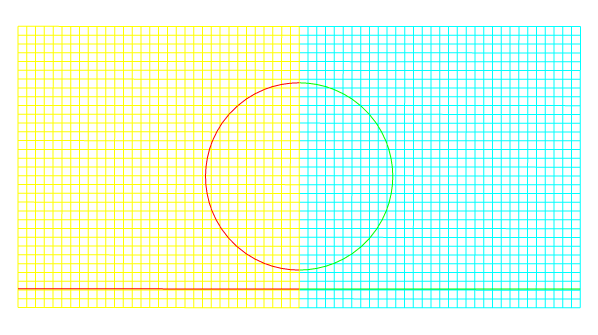# Mirror does not color lines.

#include "axi.h"
#include "fractions.h"
#include "utils.h"
#include "output.h"
#include "view.h"

scalar f[];

double geom(double x, double y) {
double C = -sq(x-0.6) - sq(y) + sq(0.5);
double L = -x;
return max(L,C);
}

int main() {
size(1.5);
origin(-0.1,0.);
init_grid (1 << 5);
fraction (f, geom(x,y));
dump (file="dump");

Then display it using Basilisk view functions.mirrored interface and cells are not colored.

  view (fov = 22.4781, quat = {0,0,0.707,-0.707},
ty = -0.43206, width = 598, height = 335);
draw_vof ("f", lc = {1,0,0} );
cells (lc = {1,1,0});
mirror (n = {0,-1,0}) {
draw_vof ("f", lc = {0,1,0});
cells (lc = {0,1,1});
}

save ("out.png");
}